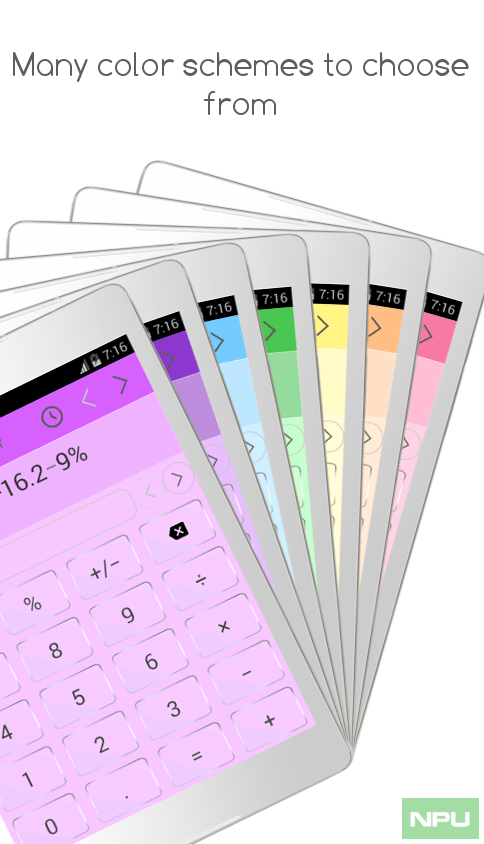The Android app which has gone free next as myAppFree app of the day is Quickey Multi Calculator Free. This is an easy calculator with multiple calculators built inside. It serves both as a scientific calculator and discount percentage calculator, but built into one single calculator app. This advanced calculator is full of functions that are suitable for many of your calculation needs.

### Quickey Multi Calculator Free Features:

* Easy scientific calculator.
* Discount percentage calculator for sale discounts along with sale tax.
* The calculator shows the work in the top area of the calculator.
* Calculator with memory plus and memory minus keys.
* Calculator with percentage key (%) to calculate percentage increase, percentage change, percentage off,…
* Decimal to fraction display of the calculation result. Proper fraction and improper fraction display modes.